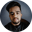Related Tags

c++
power of 2
communitycreator

# How to check if a number is a power of 2 in C++Shubham Singh Kshatriya

### Overview

In this shot, we'll see how to check if a number is a power of 2 using different approaches in C++.

### Approach 1

To check if a given number is a power of 2, we can continuously divide the number by 2, on the condition that the given number is even.

After the last possible division, if the value of the number is equal to 1, it is a power of 2. Otherwise, it is not.

### Example

#include <iostream>
using namespace std;

int main() {
int n, i;
// reading value of n from user
cin >> n;
i = n;

if (i > 0) {
// continously divide i if it is even
while (i % 2 == 0) {
i = i / 2;
}

// check if n is a power of 2
if (i == 1) {
cout << n << " is a power of 2";
} else {
cout << n << " is not a power of 2";
}
} else {
cout << "Enter a valid positive number";
}
return 0;
}

Enter the input below

### Explanation

• Line 5: We declare two variables n and i.
• Line 7–8: We read the value of n given by the user and also store it in i.
• Line 12–14: We continuously divide the value of i if it is an even number.
• Line 17–22: We check if the number n is a power of 2 by checking its value.

### Approach 2

To check if a given number is a power of 2, we can use the bit manipulation technique.

If the & operation between the given number n and given number minus one n-1 gives us 0, it is a power of 2. Otherwise, it is not.

### Example

#include <iostream>
using namespace std;

int main() {
int n;
// reading value of n from user
cin >> n;

if (n > 0) {
// & operation between n and n - 1
int i = n & (n - 1);

// check if n is a power of 2
if (i == 0) {
cout << n << " is a power of 2";
} else {
cout << n << " is not a power of 2";
}
} else {
cout << "Enter a valid positive number";
}
return 0;
}

Enter the input below

### Explanation

• Line 5: We declare a variable n.
• Line 7: We read the value of n given by the user.
• Line 11: We perform the & operation between n and n-1.
• Line 14–18: We check if the number n is a power of 2 by checking its value.

### Approach 3

To check if a given number is a power of 2, we can calculate the log2() of the given number and pass it to the ceil() and floor() methods.

If the ceil() and floor() of the passed value are equal, it is a power of 2. Otherwise, it is not.

### Example

#include <iostream>
#include <cmath>
using namespace std;

int main() {
int n;
// reading value of n from user
cin >> n;

if (n > 0) {
// calculate log2() of n
float i = log2(n);

// check if n is a power of 2
if (ceil(i) == floor(i)) {
cout << n << " is a power of 2";
} else {
cout << n << " is not a power of 2";
}
} else {
cout << "Enter a valid positive number";
}
return 0;
}

Enter the input below

### Example

• Line 6: We declare a variable n.
• Line 8: We read the value of n given by the user.
• Line 12: We calculate the log2() value of n and i is a float.
• Line 14–18: We check if the number n is a power of 2 by checking its value.

RELATED TAGS

c++
power of 2
communitycreator

CONTRIBUTORShubham Singh Kshatriya
RELATED COURSES

View all Courses

Keep Exploring

Learn in-demand tech skills in half the time